# Determine surge and swab pressure method 2

This is another method for calculating surge and swab pressure. With this method, there are 3 assumptions as follows:

• Closed ended pipe (plug flow)

• Laminar flow around drill pipe

• Turbulent flow around drill collar

How to determine surge and swab pressure with this method

1. Determine pressure around drill pipe

2. Determine pressure around drill collar

Note: The calculation methodology is different from the first method because both pressure losses are determined by separate set of equations.

3. Determine total pressure loss by summation of step 1 and step 2

4. Determine surge and swab pressure

### Determine pressure loss around drill pipe by following calculations

1. Determine fluid velocity around drill pipe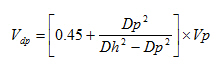Where;

Vdp is the fluid velocity around drill pipe in ft/min.

Vp is pipe movement velocity in ft/min.

Dp is drill pipe diameter in inch.

Dh is hole diameter in inch.

2. Maximum pipe velocity

Vm = Vdp x 1.5

Where;

Vdp is the fluid velocity around drill pipe in ft/min.

Vm is maximum pipe velocity.

3. Determine n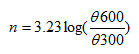Where;

n is the power law exponent.

Θ600 is a value at 600 viscometer dial reading.

Θ300 is a value at 300 viscometer dial reading.

4. Determine KWhere;

K is the fluid consistency unit.

Θ300 is a value at 300 viscometer dial reading.

n is the power law exponent.

5. Determine shear rate of drilling mud moving around drill pipe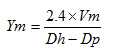Where;

Ym is shear rate of drilling mud moving around drill pipe.

Vm is maximum pipe velocity.

Dp is drill pipe diameter in inch.

Dh is hole diameter in inch.

6. Determine shear stress of drilling mud moving around drill pipe

T= K (Ym)n

Where;

T is shear stress of drilling mud moving around drill pipe.

K is the fluid consistency unit.

Ym is shear rate of drilling mud moving around drill pipe.

n is the power law exponent.

7. Determine pressure loss around drill pipeWhere;

Pdp is pressure loss around drill pipe.

T is shear stress of drilling mud moving around drill pipe.

Dp is drill pipe diameter in inch.

Dh is hole diameter in inch.

Ldp is drill pipe length in ft.

### Determine pressure loss around drill collar by following equations.

1. Determine fluid velocity around drill collar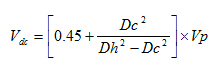Where;

Vdc is the fluid velocity around drill collar in ft/min.

Vp is pipe movement velocity in ft/min.

Dc is drill collar diameter in inch.

Dh is hole diameter in inch.

2. Maximum pipe velocity

Vm = Vdc x 1.5

Where;

Vdc is the fluid velocity around drill collar in ft/min.

Vm is maximum pipe velocity.

3. Determine equivalent flow rate in gpm due to pipe movement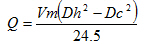Where;

Q is equivalent mud flow rate in gpm.

Vm is maximum pipe velocity.

Dc is drill collar diameter in inch.

Dh is hole diameter in inch.

4. Determine pressure loss around drill collar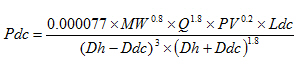Where;

Pdc is pressure loss around drill collar in psi.

MW is mud weight in ppg.

Q is equivalent mud flow rate in gpm.

PV is plastic viscosity in cp.

Ldc is length of drill collar in ft.

Dh is hole diameter in inch.

Ddc is drill collar diameter in inch.

The summation of pressure loss around drill pipe and drill collar is total pressure loss.

Total pressure loss = Pdp + Pdc

Determine surge and swab pressure with following equations:Surge pressure = Hydrostatic pressure + Total pressure lossSwab pressure = Hydrostatic pressure – Total pressure loss

Share the joy
Tagged , , , . Bookmark the permalink.1.wilson gallo says: